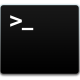## Welcome to the Treehouse Community

The Treehouse Community is a meeting place for developers, designers, and programmers of all backgrounds and skill levels to get support. Collaborate here on code errors or bugs that you need feedback on, or asking for an extra set of eyes on your latest project. Join thousands of Treehouse students and alumni in the community today. (Note: Only Treehouse students can comment or ask questions, but non-students are welcome to browse our conversations.)

### Looking to learn something new?

Treehouse offers a seven day free trial for new students. Get access to thousands of hours of content and a supportive community. Start your free trial today.``` public boolean dispense(){
boolean wasDispensed = false;
if (!isEmpty()){
pezCount--;
wasDispensed = true;
}
```Alright. lets break this down. We'll reconstruct the code above piece by piece.

First, the method has several characteristics. the public keyword declares the access level, meaning that the method is publicly accessible. the boolean keyword declares what type will be returned when the method is finished, and dispense is the name of the method. The empty parentheses after the method name indicate that no parameters have been passed into the method.

``` public boolean dispense(){
// code here was  removed for clarity
}
```

once inside the method code block, we see that the first line in the code block declares a boolean variable named wasDispensed, and sets that variable value to false.

```boolean wasDispensed = false;
// code here was removed for clarity
```

the next bit of code run after setting the wasDispensed variable is set to false is an if statement. The if statement checks a condition, and if that condition is true, then if runs the code in it's code block. We can see that the isEmpty() method is run by the if statement. However, the isEmpty() method is preceded by a logical operator of ! , which means the inverse of the declared return value. In other words, the if statement is checking if the isEmpty method returns a value of FALSE. if that is the case, it will run the if statement code in the code block.

``` public boolean dispense(){
boolean wasDispensed = false;
if (!isEmpty()){
//code to run is the method isEmpty() evaluates to false goes here
}
```

The last bit of this method is the code contained in the if statement. Lets look at the first bit.

```pezCount--;
```

The code above uses increment notation, in this case, the double minus signs, to reduce, or decrement, a variable by a value of one. So, the logic says to decrease the pezCount value by 1.

now lets look at the next bit.

```wasDispensed = true;
```

the above code is setting the wasdispensed variable to True. (remember that we have previously set this to false, right?) So, if the condition of the if statement is true, then the the dispense method will reduce the pezCount by one and change the wasDispensed variable to true.

And that is how we arrive at the code below.

``` public boolean dispense(){
boolean wasDispensed = false;
if (!isEmpty()){
pezCount--;
wasDispensed = true;
}
```

I want to know what happens when it wasDispensed = true after going inside the if statement. Why does the loop continue and how ?Good question. The answer is: The loop does not continue. In fact, there is no loop in this method (more on that in a second). Whenever we run the dispense() method, it only executes the if() statement exactly one time. The condition (in this case, !isEmpty() ) is only checked once.

Now, if we had used a 'for', 'while', or 'do...while' INSTEAD of using the 'if' statement, we would have a loop, because those statements recheck the condition argument before (or after, in the case of 'do...while' ) every execution. an 'if' statement only checks the condition once before execution.